# JP2006254226A - Acoustic signal processing apparatus, method and program, and computer-readable recording medium with acoustic signal processing program recorded thereon - Google Patents

Acoustic signal processing apparatus, method and program, and computer-readable recording medium with acoustic signal processing program recorded thereon Download PDF

## Info

Publication number
JP2006254226A
JP2006254226A JP2005069824A JP2005069824A JP2006254226A JP 2006254226 A JP2006254226 A JP 2006254226A JP 2005069824 A JP2005069824 A JP 2005069824A JP 2005069824 A JP2005069824 A JP 2005069824A JP 2006254226 A JP2006254226 A JP 2006254226A
Authority
JP
Japan
Prior art keywords
sound source
frequency
straight line
sound
acoustic signal
Prior art date
Legal status (The legal status is an assumption and is not a legal conclusion. Google has not performed a legal analysis and makes no representation as to the accuracy of the status listed.)
Granted
Application number
JP2005069824A
Other languages
Japanese (ja)
Other versions
JP3906230B2 (en
Inventor
Toshiyuki Koga
Kaoru Suzuki

Original Assignee
Toshiba Corp

Priority date (The priority date is an assumption and is not a legal conclusion. Google has not performed a legal analysis and makes no representation as to the accuracy of the date listed.)
Filing date
Publication date
Application filed by Toshiba Corp, 株式会社東芝 filed Critical Toshiba Corp
Priority to JP2005069824A priority Critical patent/JP3906230B2/en
Publication of JP2006254226A publication Critical patent/JP2006254226A/en
Application granted granted Critical
Publication of JP3906230B2 publication Critical patent/JP3906230B2/en
Application status is Expired - Fee Related legal-status Critical
Anticipated expiration legal-status Critical

• 239000002609 media Substances 0 abstract title 2
• 238000004458 analytical methods Methods 0 abstract 1
• 238000002955 isolation Methods 0 abstract 1
• 238000000034 methods Methods 0 abstract 1
• 238000009740 moulding (composite fabrication) Methods 0 abstract 1
• 230000002040 relaxant effect Effects 0 abstract 1
• 230000002123 temporal effects Effects 0 abstract 1

## Images

••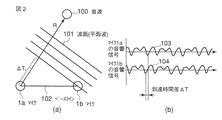••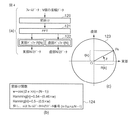•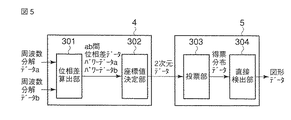•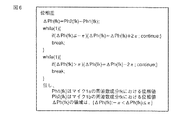•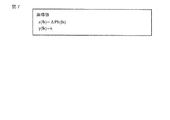•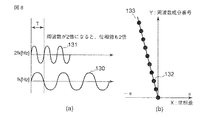•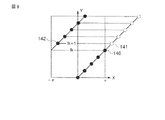•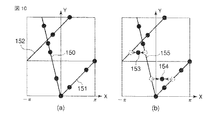•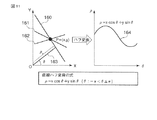•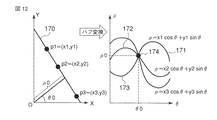•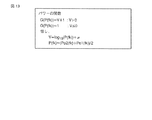•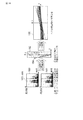•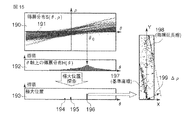•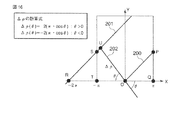•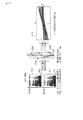•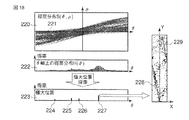•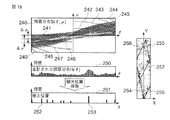••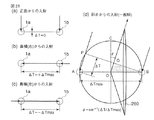•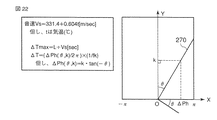•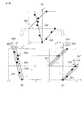•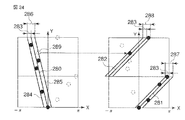••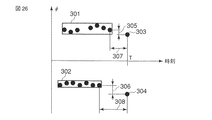••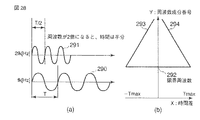•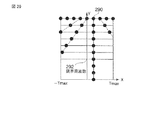•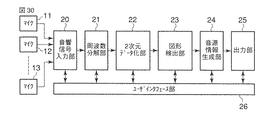•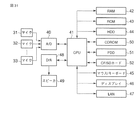•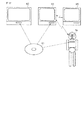## Classifications

• HELECTRICITY
• H04ELECTRIC COMMUNICATION TECHNIQUE
• H04RLOUDSPEAKERS, MICROPHONES, GRAMOPHONE PICK-UPS OR LIKE ACOUSTIC ELECTROMECHANICAL TRANSDUCERS; DEAF-AID SETS; PUBLIC ADDRESS SYSTEMS
• H04R3/00Circuits for transducers, loudspeakers or microphones
• H04R3/005Circuits for transducers, loudspeakers or microphones for combining the signals of two or more microphones
• GPHYSICS
• G10MUSICAL INSTRUMENTS; ACOUSTICS
• G10LSPEECH ANALYSIS OR SYNTHESIS; SPEECH RECOGNITION; SPEECH OR VOICE PROCESSING; SPEECH OR AUDIO CODING OR DECODING
• G10L21/00Processing of the speech or voice signal to produce another audible or non-audible signal, e.g. visual or tactile, in order to modify its quality or its intelligibility
• G10L21/02Speech enhancement, e.g. noise reduction or echo cancellation
• G10L21/0272Voice signal separating
• GPHYSICS
• G10MUSICAL INSTRUMENTS; ACOUSTICS
• G10LSPEECH ANALYSIS OR SYNTHESIS; SPEECH RECOGNITION; SPEECH OR VOICE PROCESSING; SPEECH OR AUDIO CODING OR DECODING
• G10L21/00Processing of the speech or voice signal to produce another audible or non-audible signal, e.g. visual or tactile, in order to modify its quality or its intelligibility
• G10L21/02Speech enhancement, e.g. noise reduction or echo cancellation
• G10L21/0208Noise filtering
• G10L21/0216Noise filtering characterised by the method used for estimating noise
• G10L2021/02161Number of inputs available containing the signal or the noise to be suppressed
• G10L2021/02165Two microphones, one receiving mainly the noise signal and the other one mainly the speech signal

## Abstract

An acoustic signal processing apparatus, an acoustic signal processing method, an acoustic signal processing program, and an acoustic signal processing program for sound source localization and sound source separation that can handle sound sources of more than the number of microphones while relaxing restrictions on the sound source A recording medium on which is recorded.
Two amplitude data of microphones 1a and 1b input to an acoustic signal input unit 2 are analyzed by a frequency decomposition unit 3, and a phase difference between the two is obtained for each frequency by a two-dimensional data conversion unit 4. The phase difference for each frequency is converted into two-dimensional data by giving a two-dimensional coordinate value. The graphic detector 5 detects the graphic by analyzing the generated two-dimensional data on the XY plane. The sound source information generation unit 6 processes the detected graphic information, and the number of sound sources that are the sources of acoustic signals, the spatial existence range of each sound source, the temporal existence period of the sound emitted by each sound source, and each sound source Sound source information including a sound component configuration, separated sound for each sound source, and symbolic contents of each sound source sound is generated.
[Selection] Figure 1

## Description

The present invention relates to acoustic signal processing, and more particularly to estimation of the number of sound wave sources that have propagated through a medium, the direction of each source, the frequency components of sound waves that arrive from each source, and the like.

In recent years, in the field of auditory research for robots, a method has been proposed in which the number and direction of multiple target sound sources are estimated in a noisy environment (sound source localization), and each sound source sound is separated and extracted (sound source separation). .

For example, in Non-Patent Document 1 below, N sound source sounds are observed with M microphones in an environment with background noise, and a spatial correlation matrix is generated from data obtained by subjecting each microphone output to a short-time Fourier transform (FFT) process. A method of estimating the number N of sound sources as the number of main eigenvalues by decomposing this into eigenvalues and obtaining main eigenvalues having a large value is described. This utilizes the property that a directional signal such as a sound source is mapped to main eigenvalues, and background noise having no directionality is mapped to all eigenvalues. The eigenvector corresponding to the main eigenvalue is a base vector of the signal subspace spanned by the signal from the sound source, and the eigenvector corresponding to the remaining eigenvalue is the base vector of the noise subspace spanned by the background noise signal. By applying the MUSIC method using the basis vector of this noise subspace, the position vector of each sound source can be searched, and the sound source can be obtained with a beamformer having directivity in the direction obtained as a result of the search. The voice from can be extracted. However, when the number N of sound sources is the same as the number M of microphones, the noise subspace cannot be defined, and when the number N of sound sources exceeds M, there are sound sources that cannot be detected. Therefore, the number of sound sources that can be estimated does not exceed the number M of microphones. This method has no particular limitation on the sound source, and is a mathematically clean method. However, in order to handle a large number of sound sources, there is a limitation that more microphones are required.

Non-Patent Document 2 below describes a method of performing sound source localization and sound source separation using a pair of microphones. This method pays attention to the harmonic structure (frequency structure consisting of fundamental frequency and its harmonics) peculiar to the sound generated through the tube (articulator) like human voice, and the sound signal captured by the microphone By detecting harmonic structures with different fundamental frequencies from the Fourier-transformed data, the number of detected harmonic structures is the number of speakers, and the interaural phase difference (IPD) and binaural for each harmonic structure. The direction is estimated with certainty using the intensity difference (IID), and each sound source sound is estimated by the harmonic structure itself. This method can process more than the number of microphones by detecting a plurality of harmonic structures from Fourier transform data. However, since the number and direction of sound sources and the basis of sound source sound estimation are based on harmonic structures, the sound sources that can be handled are limited to those with harmonic structures like human voices, and can be used for various sounds. It cannot be handled.
Asano Tai, "Dividing Sounds", Measurement and Control, Vol. 43, No. 4, pp. 325-330, April 2004 issue Nakahiro Kazuhiro et al., "Real-Time Active Person Tracking by Hierarchical Integration of Audiovisual Information", AI Society AI Challenge Study Group, SIG-Challenge-0113-5, pp. 35-42, June 2001

As described above, (1) the number of sound sources cannot be greater than the number of microphones if there are no restrictions on the sound source, and (2) when the number of sound sources is greater than the number of microphones, for example, a harmonic structure is assumed for the sound source. There is a contradictory problem that there are restrictions such as the ability to do so, and no method has been established that can handle sound sources more than the number of microphones without restricting the sound sources.

The present invention has been made in view of the above-described problems, further relaxes restrictions on sound sources, and can handle sound sources with more than the number of microphones, and an acoustic signal processing device for sound source localization and sound source separation, An object of the present invention is to provide an acoustic signal processing method, an acoustic signal processing program, and a computer-readable recording medium on which the acoustic signal processing program is recorded.

An acoustic signal processing apparatus according to an aspect of the present invention is an acoustic signal input unit that inputs a plurality of acoustic signals captured at two or more points that are not spatially identical, and decomposes each of the plurality of acoustic signals, A frequency resolving means for obtaining a plurality of frequency resolved data sets representing phase values for each frequency; a phase difference calculating means for calculating a phase difference value for each frequency in different sets of the plurality of frequency resolved data sets; A two-dimensional representation of a point group having a coordinate value on a two-dimensional coordinate system with a frequency function as a first axis and a phase difference value function calculated by the phase difference calculation means as a second axis. A two-dimensional data generating means for generating data, a graphic detecting means for detecting a graphic reflecting a proportional relationship between a frequency and a phase difference derived from the same sound source from the two-dimensional data, and a source of the acoustic signal The number of sound sources to be hit, the spatial existence range of each sound source, the temporal existence period of the sound emitted by each sound source, the component composition of the sound emitted by each sound source, the separated sound separated for each sound source, Acoustic signal processing comprising: sound source information generating means for generating sound source information relating to a distinguished sound source including at least one of the symbolic contents of the emitted sound based on the graphic; and output means for outputting the sound source information Device.

According to the present invention, an acoustic signal processing device, an acoustic signal processing method, an acoustic signal processing program for sound source localization and sound source separation that can further ease restrictions on a sound source and can handle sound sources of more than the number of microphones, And a computer-readable recording medium on which the acoustic signal processing program is recorded.

DESCRIPTION OF EMBODIMENTS Hereinafter, embodiments of an acoustic signal processing device according to the present invention will be described with reference to the drawings.

FIG. 1 is a functional block diagram of an acoustic signal processing apparatus according to an embodiment of the present invention. This acoustic signal processing apparatus includes a microphone 1a, a microphone 1b, an acoustic signal input unit 2, a frequency decomposition unit 3, a two-dimensional data conversion unit 4, a graphic detection unit 5, a sound source information generation unit 6, and an output. Unit 7 and a user interface unit 8.

[Basic concept of sound source estimation based on phase difference for each frequency component]
The microphone 1a and the microphone 1b are two microphones arranged at a predetermined distance in a medium such as air, and convert medium vibrations (sound waves) at two different points into electric signals (acoustic signals), respectively. It is means of. Hereinafter, when the microphone 1a and the microphone 1b are collectively handled, they are referred to as a microphone pair.

The acoustic signal input unit 2 periodically A / D-converts two acoustic signals from the microphone 1a and the microphone 1b at a predetermined sampling period Fr, thereby digitizing the two acoustic signals from the microphone 1a and the microphone 1b. This is means for generating amplitude data in time series.

Assuming that the sound source is located far enough from the distance between the microphones, as shown in FIG. 2A, the wavefront 101 of the sound wave emitted from the sound source 100 and reaching the microphone pair is substantially flat. . When this plane wave is observed at two different points by using the microphone 1a and the microphone 1b, the microphone is selected according to the direction R of the sound source 100 with respect to a line segment 102 (referred to as a baseline) connecting the microphone 1a and the microphone 1b. A predetermined arrival time difference ΔT should be observed in the acoustic signals converted in pairs. When the sound source is sufficiently far away, the arrival time difference ΔT becomes 0 when the sound source 100 exists on a plane perpendicular to the baseline 102, and this direction is defined as the front direction of the microphone pair.

Reference 1 “Satoshi Suzuki et al.,“ Realization of “calling” function of home robot by audio-visual cooperation ””, Proc. Of the 4th SICE System Integration Division Annual Conference (SI2003), 2F4-5, 2003 ” In this case, by searching which part of one amplitude data is similar to which part of the other amplitude data by pattern matching, between two acoustic signals (103 and 104 in FIG. 2 (b)) A method for deriving the arrival time difference ΔT is described. However, this method is effective when there is only one strong sound source, but when there is strong background noise or multiple sound sources, similar parts are clearly shown on the waveform containing strong sounds from multiple directions. It may not appear and pattern verification may fail.

Therefore, in the present invention, the input amplitude data is decomposed into a phase difference for each frequency component and analyzed. When there are a plurality of sound sources, a phase difference corresponding to the sound source direction is observed between the two data for the frequency components of each sound source. Therefore, if the phase difference of each frequency component can be divided into groups in the same direction without assuming strong constraints on the sound source, there will be several sound sources for a wider variety of sound sources, each of which direction in which direction. It should be possible to grasp how each of them emits a sound wave having a characteristic frequency component. The reasoning itself is very simple and clear, but there are some challenges to overcome when analyzing actual data. Along with this problem, functional blocks (frequency decomposition unit 3, two-dimensional data conversion unit 4, and figure detection unit 5) for performing this grouping will be described.

[Frequency decomposition unit 3]
As a general technique for decomposing amplitude data into frequency components, there is a fast Fourier transform (FFT). As a typical algorithm, a Cooley-Turkey DFT algorithm and the like are known.

As shown in FIG. 3, the frequency resolving unit 3 extracts N pieces of continuous amplitude data as frames (T-th frame 111) from the amplitude data 110 obtained by the acoustic signal input unit 2 and performs fast Fourier transform. This extraction position is repeated while shifting the frame shift amount by 113 (T + 1th frame 112).

The amplitude data constituting the frame is subjected to windowing 120 as shown in FIG. As a result, short-time Fourier transform data of the input frame is generated in the real part buffer R [N] and the imaginary part buffer I [N] (122). An example of the windowing function (Hamming windowing or Hanning windowing) 124 is shown in FIG.

The short-time Fourier transform data generated here is data obtained by decomposing the amplitude data of the frame into N / 2 frequency components, and the real part R [k] in the buffer 122 for the kth frequency component fk. The numerical value of the imaginary part I [k] represents the point Pk on the complex coordinate system 123 as shown in FIG. The square of the distance from the origin O of Pk is the power Po (fk) of the frequency component, and the signed rotation angle θ {θ: −π> θ ≧ π [radian]} from the real part axis of Pk is This is the phase Ph (fk) of the frequency component.

When the sampling frequency is Fr [Hz] and the frame length is N [sample], k takes an integer value from 0 to (N / 2) −1, k = 0 is 0 [Hz] (DC), k = (N / 2) -1 represents Fr / 2 [Hz] (highest frequency component), and the frequency resolution Δf = (Fr / 2) ÷ ((N / 2) -1) [Hz] is equally divided between them. This is the frequency at each k and is expressed by fk = k · Δf.

As described above, the frequency resolving unit 3 continuously performs this processing with a predetermined interval (frame shift amount Fs), thereby making it possible to generate a frequency composed of a power value and a phase value for each frequency of the input amplitude data. Generate decomposition data set in time series.

(Two-dimensional data conversion unit 4 and figure detection unit 5)
As shown in FIG. 5, the two-dimensional data conversion unit 4 includes a phase difference calculation unit 301 and a coordinate value determination unit 302. The figure detection unit 5 includes a voting unit 303 and a straight line detection unit 304.

[Phase difference calculation unit 301]
The phase difference calculation unit 301 is obtained by comparing two frequency resolution data sets a and b at the same time obtained by the frequency resolution unit 3 and calculating the difference between the phase values for each same frequency component. This is means for generating inter-ab phase difference data. For example, as shown in FIG. 6, the phase difference ΔPh (fk) of a certain frequency component fk is calculated by calculating the difference between the phase value Ph1 (fk) at the microphone 1a and the phase value Ph2 (fk) at the microphone 1b. It is calculated as a 2π residue system so that {ΔPh (fk): −π <ΔPh (fk) ≦ π}.

[Coordinate value determination unit 302]
The coordinate value determination unit 302 calculates the phase difference data obtained by calculating the difference between both phase values for each frequency component based on the phase difference data obtained by the phase difference calculation unit 301, and outputs a predetermined two-dimensional XY coordinate. It is a means for determining coordinate values to be handled as points on the system. The X coordinate value x (fk) and the Y coordinate value y (fk) corresponding to the phase difference ΔPh (fk) of a certain frequency component fk are determined by the equations shown in FIG. The X coordinate value is the phase difference ΔPh (fk), and the Y coordinate value is the frequency component number k.

[Frequency proportionality of phase difference to same time difference]
The phase difference for each frequency component calculated by the phase difference calculation unit 301 as shown in FIG. 6 should represent the same arrival time difference between those derived from the same sound source (in the same direction). The phase value of a certain frequency obtained by FFT and the phase difference between the microphones are values calculated with the frequency period being 2π. Here, even if the time difference is the same, attention is paid to the existence of a proportional relationship in which the phase difference also doubles when the frequency doubles. This is shown in FIG. As shown in FIG. 8A, for the same time T, the wave 130 with the frequency fk [Hz] includes a half period, that is, a phase section of π, but the wave 131 with the double frequency 2fk [Hz]. Includes one period, that is, a phase interval of 2π. The same applies to the phase difference. That is, for the same time difference ΔT, the phase difference increases in proportion to the frequency. FIG. 8B shows the proportional relationship between the phase difference and the frequency. When the phase difference of each frequency component emitted from the same sound source and having a common ΔT is plotted on the two-dimensional coordinate system by the coordinate value calculation shown in FIG. 7, the coordinate point 132 representing the phase difference of each frequency component is a straight line 133. You can see that they line up on top. The greater the ΔT, that is, the greater the distance to the sound source, the greater the slope of this straight line.

[Circulation of phase difference]
However, as shown in FIG. 8B, the phase difference between the microphones is proportional to the frequency throughout the range when the true phase difference does not deviate from ± π from the lowest frequency to the highest frequency to be analyzed. Limited to. This condition is that ΔT does not become equal to or longer than the time corresponding to ½ period of the maximum frequency (half the sampling frequency) Fr / 2 [Hz], that is, 1 / Fr [second]. If ΔT is 1 / Fr or more, it must be considered that the phase difference can only be obtained as a cyclic value as described below.

The phase value for each frequency component can be obtained only as a value of the rotation angle θ shown in FIG. 4 with a width of 2π (in this embodiment, a width of 2π between −π and π). This means that even if the actual phase difference in the frequency component is one period or more between the microphones, it cannot be known from the phase value obtained as a result of frequency decomposition. Therefore, in the present embodiment, the phase difference is obtained between −π and π as shown in FIG. However, the true phase difference caused by ΔT may be a value obtained by adding or subtracting 2π to the value of the phase difference obtained here, or adding or subtracting 4π or 6π. This is schematically shown in FIG. In FIG. 9, when the phase difference ΔPh (fk) of the frequency fk is + π as represented by the black circle 140, the phase difference of the next higher frequency fk + 1 exceeds + π as represented by the white circle 141. However, the calculated phase difference ΔPh (fk + 1) is slightly larger than −π as indicated by the black circle 142 obtained by subtracting 2π from the original phase difference. Although not shown, a similar value is shown even at a frequency three times that, but this is a value obtained by subtracting 4π from the actual phase difference. Thus, the phase difference circulates between −π and π as a 2π residue system as the frequency increases. As shown in this example, when ΔT increases, the true phase difference represented by a white circle circulates on the opposite side as indicated by the black circle above a certain frequency fk + 1.

[Phase difference when multiple sound sources are present]
On the other hand, when sound waves are emitted from a plurality of sound sources, the plot of the frequency and phase difference is as shown schematically in FIG. This figure shows a case where two sound sources exist in different directions with respect to the microphone pair, and FIG. 10A shows a case where the two sound source sounds do not contain the same frequency component. FIG. 10B shows a case where some frequency components are included in both. In FIG. 10A, the phase difference of each frequency component is on one of the straight lines having the same ΔT. The straight line 150 with a small slope has five points, and the straight line 151 with a large slope (including the circulated straight line 152). Then, 6 points are arranged on a straight line. In FIG. 10 (b), the two frequency components 153 and 154 included in both are mixed with waves and the phase difference does not appear correctly. Therefore, it does not ride on any straight line, and only the three straight lines 155 have a particularly small slope. Not on the top.

The problem of estimating the number and direction of sound sources can be reduced to finding a straight line as shown on such a plot. Further, the problem of estimating the frequency component for each sound source can be reduced to selecting a frequency component arranged at a position close to the detected straight line. In the present embodiment, the two-dimensional data output from the two-dimensional data conversion unit 4 is a point group determined as a function of frequency and phase difference using two of the frequency decomposition data sets by the frequency decomposition unit 3, or those points. An image in which the group is arranged (plotted) on a two-dimensional coordinate system is used. Note that this two-dimensional data is defined by two axes that do not include a time axis. Therefore, three-dimensional data as a time series of two-dimensional data can be defined. The figure detection unit 5 detects a linear arrangement as a figure from the point group arrangement given as the two-dimensional data (or three-dimensional data as time series thereof).

[Voting section 303]
The voting unit 303 applies a linear Hough transform to each frequency component given the (x, y) coordinates by the coordinate value determining unit 302 as described later, and the trajectory in the Hough voting space by a predetermined method. A means to vote. The Hough transform is explained on pages 100 to 102 of Reference Document 2 “Akio Okazaki,“ Initial Image Processing ”, Industrial Research Committee, Issued on October 20, 2000”. To do.

[Linear Hough Transform]
As schematically shown in FIG. 11, there are an infinite number of straight lines that can pass through the point p (x, y) on the two-dimensional coordinates as illustrated in 160, 161, and 162. If the inclination of the vertical line 163 from the X axis is expressed as θ and the length of the vertical line 163 is expressed as ρ, θ and ρ are uniquely determined for one straight line. It is known that a set of θ and ρ that can be taken by a straight line passing through a point (x, y) draws a trajectory 164 (ρ = x cos θ + ysin θ) specific to the value of (x, y) on the θρ coordinate system. Yes. Such conversion from the (x, y) coordinate value to the locus of (θ, ρ) of a straight line passing through (x, y) is called a straight line Hough transform. Note that θ is a positive value when the straight line is tilted to the left, 0 when it is vertical, and a negative value when it is tilted to the right, and the definition range of θ is {θ: −π <θ ≦ π. } Is not deviated.

Although the Hough curve can be obtained independently for each point on the XY coordinate system, as shown in FIG. 12, for example, a straight line 170 that passes through the three points p1, p2, and p3 in common corresponds to p1, p2, and p3. It can be obtained as a straight line defined by the coordinates (θ0, ρ0) of the point 174 where the trajectories 171, 172, 173 intersect. The more lines that pass through the points, the more trajectories pass through the positions of θ and ρ representing the lines. Thus, the Hough transform is suitable for use in detecting a straight line from a point group.

[Hough voting]
An engineering technique called Hough voting is used to detect straight lines from point clouds. This is because by voting a set of θ and ρ through which each trajectory passes in a two-dimensional Hough voting space with θ and ρ as coordinate axes, θ and ρ through which a large number of trajectories pass at a large position in the Hough voting space. This is a technique for suggesting the existence of a pair, that is, a straight line. In general, a two-dimensional array (Hough voting space) having a size corresponding to a necessary search range for θ and ρ is first prepared and initialized to zero. Next, the trajectory for each point is obtained by Hough transform, and the value on the array through which this trajectory passes is incremented by one. This is called Hough voting. When voting for the trajectory for all points is completed, there is no straight line at the position of vote 0 (no trajectory has passed), and 1 at the position of vote 1 (only one trajectory has passed). A straight line passing through two points has a straight line passing through two points at the position of vote 2 (only two trajectories have passed), and n points at a position of vote n (only n trajectories have passed). It can be seen that there are straight lines that pass through. If the resolution of the Hough voting space can be made infinite, as described above, only the points that the trajectory passes will obtain the votes corresponding to the number of trajectories passing through it, but the actual Hough voting space is about θ and ρ. Since it is quantized with an appropriate resolution, a high vote distribution is generated around the position where a plurality of trajectories intersect. Therefore, it is necessary to more accurately obtain the position where the trajectory intersects by searching for the position having the maximum value from the vote distribution in the Hough voting space.

The voting unit 303 performs Hough voting on frequency components that satisfy all of the following voting conditions. Under this condition, only frequency components having a power equal to or higher than a predetermined threshold in a predetermined frequency band are voted.

In other words, the voting condition 1 is that the frequency is in a predetermined range (low frequency cut and high frequency cut). The voting condition 2 is that the power P (fk) of the frequency component fk is equal to or greater than a predetermined threshold value.

The voting condition 1 is generally used for the purpose of cutting a low range where background noise is riding or cutting a high range where the accuracy of FFT is reduced. The range of the low frequency cut and the high frequency cut can be adjusted according to the operation. When using the widest frequency band, it is appropriate to set the low frequency cut to only the DC component and the high frequency cut to the maximum frequency only.

It is considered that the reliability of the FFT result is not high with a very weak frequency component such as background noise. The voting condition 2 is used for the purpose of preventing such frequency components having low reliability from participating in voting by thresholding with power. Assuming that the power value Po1 (fk) in the microphone 1a and the power value Po2 (fk) in the microphone 1b, there are three possible ways of determining the power P (fk) evaluated at this time. Which condition is used can be set according to the operation.

(Average value): The average value of Po1 (fk) and Po2 (fk). This is a condition that requires both powers to be reasonably strong.

(Minimum value): The smaller of Po1 (fk) and Po2 (fk). This is a condition that requires both powers to be at least a threshold value.

(Maximum value): The larger of Po1 (fk) and Po2 (fk). The condition is that if one is less than the threshold but the other is strong enough to vote.

The voting unit 303 can perform the following two addition methods when voting.

That is, in addition method 1, a predetermined fixed value (for example, 1) is added to the trajectory passing position. In the addition method 2, the function value of the power P (fk) of the frequency component fk is added to the trajectory passing position.

The addition method 1 is a method that is generally used in the straight line detection problem by the Hough transform, and since the votes are ranked in proportion to the number of passing points, a straight line including many frequency components (that is, It is suitable for preferential detection of sound sources. At this time, since there is no limitation on the harmonic structure (the included frequencies are equally spaced) for the frequency components included in the straight line, it is possible to detect a wider variety of sound sources, not limited to human speech.

In addition, the addition method 2 is a method in which even if there are few points to pass through and a frequency component with high power is included, a higher maximum value can be obtained. It is suitable for detecting a straight line (that is, a sound source) having The function value of the power P (fk) in the addition method 2 is calculated as G (P (fk)). FIG. 13 shows a calculation formula for G (P (fk)) when P (fk) is an average value of Po1 (fk) and Po2 (fk). In addition to the above voting condition 2, it is also possible to calculate P (fk) as the minimum and maximum values of Po1 (fk) and Po2 (fk). Can be set. The value of the intermediate parameter V is calculated as a value obtained by adding a predetermined offset α to the logarithmic value log 10 (P (fk)) of P (fk). When V is positive, the value of V + 1 is set as the value of the function G (P (fk)). In this way, by voting at least 1, not only a straight line (sound source) containing a high frequency component rises to the top, but also a straight line (sound source) containing a number of frequency components rises to the top. It is possible to have one majority property. The voting unit 303 can perform either the addition method 1 or the addition method 2 depending on the setting, but in particular, by using the latter, it becomes possible to simultaneously detect a sound source with a small frequency component, and a wider variety of types. The sound source can be detected.

[Voting multiple FFT results together]
Further, the voting unit 303 can vote for each FFT, but generally, the voting unit 303 collectively votes for m consecutive (m ≧ 1) time-series FFT results. In the long term, the frequency components of the sound source will fluctuate, but by doing so, using more data obtained from the FFT results of moderately short time multiple times where the frequency components are stable You will be able to get a more reliable Hough voting result. Note that m can be set as a parameter according to the operation.

[Linear detection unit 304]
The straight line detection unit 304 is a means for analyzing the vote distribution in the Hough voting space generated by the voting unit 303 and detecting a powerful straight line. However, at this time, more accurate straight line detection is realized by taking into consideration the circumstances peculiar to this problem, such as the phase difference circulation described in FIG.

FIG. 14 shows the power spectrum of the frequency component when processing is performed using an actual voice spoken from the left of the front of the microphone pair by about 20 degrees in the room noise environment, for five consecutive times (m = 5) A phase difference plot diagram for each frequency component obtained from the FFT result, and a Hough vote result (voting distribution) obtained from the same five FFT results. The processing so far is executed by a series of functional blocks from the acoustic signal input unit 2 to the voting unit 303.

Amplitude data acquired by the microphone pair is converted into power value and phase value data for each frequency component by the frequency resolving unit 3. In FIG. 14, 180 and 181 indicate the logarithm of the power value for each frequency component with the horizontal axis as time, and the luminance display (larger as black). FIG. 5 is a diagram in which one vertical line corresponds to one FFT result and is graphed over time (rightward). The upper stage 180 is the result of processing the signal from the microphone 1a and the lower stage 181 is the signal from the microphone 1b, and a large number of frequency components are detected. In response to this frequency decomposition result, the phase difference calculation unit 301 obtains a phase difference for each frequency component, and the coordinate value decision unit 302 calculates the (x, y) coordinate value. In FIG. 14, 182 is a diagram in which the phase difference obtained by FFT for five consecutive times from a certain time 183 is plotted. In this figure, a point cloud distribution along the straight line 184 tilted to the left from the origin is recognized, but the distribution is not neatly placed on the straight line 184, and there are many points apart from the straight line 184. Yes. Each point indicating such distribution is voted by the voting unit 303 to the Hough voting space to form a vote distribution 185. In the figure, reference numeral 185 denotes a vote distribution generated using the addition method 2.

[Constraint of ρ = 0]
By the way, when the signals of the microphone 1a and the microphone 1b are A / D converted in phase by the acoustic signal input unit 2, the straight line to be detected always passes through ρ = 0, that is, the origin of the XY coordinate system. Therefore, the sound source estimation problem results in a problem of searching for a maximum value from the vote distribution S (θ, 0) on the θ axis where ρ = 0 in the Hough voting space. FIG. 15 shows the result of searching for the maximum value on the θ-axis with respect to the data illustrated in FIG.

In FIG. 15, the vote distribution 190 is the same as the vote distribution 185 in FIG. The bar graph 192 is obtained by extracting the vote distribution S (θ, 0) on the θ axis 191 as H (θ). This vote distribution H (θ) has several local maximum points (protrusions). When the straight line detection unit 304 searches for the vote distribution H (θ) as long as (1) the same point as itself continues for a certain position on the left and right, only the one with the lower vote than the last appears. Leave. As a result, the local maximum on the vote distribution H (θ) is extracted. Since the local maximum includes a portion having a flat peak, the local maximum is continuous there. Therefore, the straight line detection unit 304 leaves only the center position of the maximum portion as the maximum position 193 by (2) thinning processing. Finally, (3) only the maximum position where the vote is equal to or greater than a predetermined threshold is detected as a straight line. In this way, it is possible to accurately determine θ of a straight line obtained with a sufficient vote. In the example of the figure, among the maximum positions 194, 195, and 196 detected in (2) above, the maximum position 194 is the center position left by the thinning process from the flat maximum portion (the right is given priority when even numbers are continuous). It is. Further, only 196 is a straight line detected by obtaining a vote exceeding the threshold. A straight line (reference straight line) 197 is defined by θ and ρ (= 0) given by the local maximum position 196. As the thinning algorithm, the “Tamura method” described on pages 89 to 92 of Reference 2 referred to in the description of the Hough transform can be used in a one-dimensional manner. When the straight line detection unit 304 detects one or a plurality of maximum positions (the center position where the votes obtained above a predetermined threshold) are detected in this way, the straight line detection unit 304 ranks them in descending order of the votes and determines the values of θ and ρ at each maximum position. Is output.

[Definition of straight line group considering phase difference circulation]
Incidentally, the straight line 197 illustrated in FIG. 15 is a straight line passing through the XY coordinate origin defined by the local maximum position 196 of (θ0, 0). However, the straight line 198 in which the straight line 197 in FIG. 15 is translated by Δρ199 and circulates from the opposite side on the X axis due to the cyclic nature of the phase difference is also a straight line showing the same arrival time difference as the 197. Like this straight line 198, the straight line 197 is extended and the part protruding from the X value range appears cyclically from the opposite side as the “circular extension line” of the straight line 197, and the reference straight line 197 is the “reference straight line”. I will call them respectively. If the reference straight line 197 is further inclined, the circulation extension line is further increased in number. If the coefficient a is an integer greater than or equal to 0, all straight lines having the same arrival time difference are a straight line group (θ0, aΔρ) obtained by translating the reference straight line 197 defined by (θ0, 0) by Δρ. Further, when the starting point ρ is generalized as ρ = ρ0 by removing the restriction of ρ = 0, the straight line group can be described as (θ0, aΔρ + ρ0). At this time, Δρ is a signed value defined by the equation shown in FIG. 16 as a function Δρ (θ) of the slope θ of the straight line.

In FIG. 16, the reference straight line 200 is defined by (θ, 0). At this time, since the reference straight line 200 is inclined to the right, θ is a negative value according to the definition, but is treated as an absolute value in the figure. A straight line 201 in FIG. 16 is a circulation extension line of the reference straight line 200, and intersects the X axis at the point R. The interval between the reference straight line 200 and the circulation extension line 201 is Δρ as shown by the auxiliary line 202, and the auxiliary line 202 intersects the reference straight line 200 perpendicularly at the point O and intersects the circulation extension line 201 perpendicularly at the point U. is doing. At this time, since the reference straight line is tilted to the right, Δρ is also a negative value according to the definition, but is treated as an absolute value in the figure. In FIG. 16, ΔOQP is a right triangle whose side OQ has a length of π, and a triangle congruent with this is ΔRTS. Therefore, it can be seen that the length of the side RT is also π, and the length of the hypotenuse OR of ΔOUR is 2π. At this time, since Δρ is the length of the side OU, Δρ = 2πcos θ. Then, taking the signs of θ and Δρ into consideration, the calculation formula of the figure is derived.

[Maximum position detection considering phase difference circulation]
From the circulation of the phase difference, it was stated that the straight line representing the sound source should be treated as a straight line group consisting of a reference straight line and a circulation extension line instead of one. This must be taken into account when detecting the maximum position from the vote distribution. Usually, the vote value on ρ = 0 (or ρ = ρ0) (that is, the reference) is limited to the case where the sound source is detected only in the vicinity of the front of the microphone pair that does not circulate in the phase difference or is small even if it occurs. The above-described method of searching for a maximal position using only a straight vote value is sufficient in terms of performance, and is effective in shortening the search time and improving accuracy. However, in order to detect a sound source that exists in a wider range, it is necessary to search for a maximum position by summing up several vote values separated by Δρ for a certain θ. This difference is explained below.

FIG. 17 shows the power spectrum of the frequency component for five times when processing is performed using actual voices simultaneously spoken from about 20 degrees left and about 45 degrees right in front of the microphone pair in a room noise environment. The phase difference plot figure for every frequency component obtained from the FFT result of m = 5), and the Hough vote result (voting distribution) obtained from the same five FFT results are shown.

Amplitude data acquired by the microphone pair is converted into power value and phase value data for each frequency component by the frequency resolving unit 3. In FIG. 17, reference numerals 210 and 211 indicate the logarithm of the power value for each frequency component, with the frequency on the vertical axis and the time on the horizontal axis, in luminance display (larger in black). FIG. 5 is a diagram in which one vertical line corresponds to one FFT result and is graphed over time (rightward). The upper stage 210 is the result of processing the signal from the microphone 1a and the lower stage 211 is the signal from the microphone 1b, and a large number of frequency components are detected. In response to this frequency decomposition result, the phase difference calculation unit 301 obtains a phase difference for each frequency component, and the coordinate value decision unit 302 calculates the (x, y) coordinate value. The plot diagram 212 is a plot of phase differences obtained by FFT for five consecutive times from a certain time 213. In this plot diagram 212, a point cloud distribution along the reference line 214 inclined to the left from the origin and a point cloud distribution along the reference line 215 inclined to the right are recognized. Each point indicating such a distribution is voted by the voting unit 303 in the Hough voting space to form a vote distribution 216. The vote distribution 216 is generated using the addition method 2.

FIG. 18 is a diagram showing a result of searching for the maximum position only by the vote value on the θ axis. The vote distribution 220 in FIG. 18 is the same as the vote distribution 216 in FIG. The bar graph 222 is a bar graph obtained by extracting the vote distribution S (θ, 0) on the θ axis 221 as H (θ). In this vote distribution H (θ), there are several local maximum points (protrusions), but as a whole, it can be seen that the larger the absolute value of θ, the smaller the votes. From this vote distribution H (θ), as shown in the maximum position graph 223, four maximum positions 224, 225, 226, and 227 are detected. Among these, only the maximum position 227 obtains a vote that is equal to or greater than the threshold value. Thereby, one straight line group (reference straight line 228 and circulation extension line 229) is detected. This straight line group is a sound detected from the left about 20 degrees in front of the microphone pair, but a sound from the right about 45 degrees in front of the microphone pair cannot be detected. Since the reference straight line passing through the origin can pass only a small frequency band before the value range of X is exceeded as the angle is larger, the width of the frequency band through which the reference straight line varies depends on θ (unfair). Since the constraint of ρ = 0 causes the votes for only the reference line to compete under this unfair condition, the straight line with a larger angle is disadvantageous for the vote. This is the reason why the voice from the right about 45 degrees could not be detected.

On the other hand, FIG. 19 is a diagram showing the result of searching for the maximum position by adding the vote values at several places separated by Δρ. In the figure, 240 indicates the position of ρ as indicated by broken lines 242-249 when the straight line passing through the origin is translated by Δρ on the vote distribution 216 in FIG. At this time, the θ axis 241 and the broken lines 242 to 245 and the θ axis 241 and the broken lines 246 to 249 are spaced apart at equal intervals by a natural number multiple of Δρ (θ). It should be noted that there is no broken line at θ = 0 where it is certain that the straight line will pass through the ceiling of the plot diagram without exceeding the X value range.

A given vote H (θ0) of θ0 is the total value of the votes on the θ-axis 241 and the votes on the broken lines 242-249 when viewed vertically at the position θ = θ0, that is, H (θ0) = Σ {S ( θ0, aΔρ (θ0))}. This operation is equivalent to adding up the votes of the reference straight line where θ = θ0 and the circulation extension line. A bar graph representing the vote distribution H (θ) is 250 in the figure. Unlike 222 in FIG. 18, in this distribution, the number of votes does not decrease even when the absolute value of θ increases. This is because the same frequency band can be used for all θ by adding a circulation extension line to the vote calculation. From this vote distribution 250, ten maximum positions indicated by 251 in the figure are detected. Among these, a straight line group (reference straight line 254 and circulation extension line 255 corresponding to the maximum position 253), in which the maximum positions 252 and 253 have obtained a vote equal to or greater than the threshold and the sound from the left of the front of the microphone pair about 20 degrees is detected; Two groups of straight lines (reference line 256 corresponding to the maximum position 252 and circulation extension lines 257 and 258) that detect the sound from the right about 45 degrees in front of the microphone pair are detected. In this way, by searching for the maximum position by summing the vote values at locations separated by Δρ, it becomes possible to detect stably from a straight line with a small angle to a straight line with a large angle.

[Maximum position detection considering non-in-phase case: Generalization]
When the signals of the microphone 1a and the microphone 1b are not A / D converted in phase by the acoustic signal input unit 2, the straight line to be detected does not pass through ρ = 0, that is, the XY coordinate origin. In this case, it is necessary to search for the maximum position by removing the constraint of ρ = 0.

If the reference straight line from which the constraint of ρ = 0 is removed and generalized and described as (θ0, ρ0), the straight line group (reference straight line and circulation extension line) can be described as (θ0, aΔρ (θ0) + ρ0). Here, Δρ (θ0) is a parallel movement amount of the circulation extension line determined by θ0. When the sound source comes from a certain direction, there is only one of the most powerful straight line groups corresponding to θ0. The straight line group is obtained by using the value ρ0max of ρ0 that maximizes the Σ {S (θ0, aΔρ (θ0) + ρ0)} of the straight line group when ρ0 is changed variously (θ0, aΔρ (θ0) + ρ0max). Given in. Therefore, the same maximum position detection algorithm as when ρ = 0 is applied by applying the vote H (θ) at each θ to the maximum vote value Σ {S (θ, aΔρ (θ) + ρ0max)} at each θ. Straight line detection can be performed.

The number of straight line groups detected in this way is the number of sound sources.

[Sound source information generation unit 6]
As illustrated in FIG. 20, the sound source information generation unit 6 includes a direction estimation unit 311, a sound source component estimation unit 312, a sound source sound resynthesis unit 313, a time series tracking unit 314, a duration evaluation unit 315, 316, an adaptive array processing unit 317, and a speech recognition unit 318.

[Direction estimation unit 311]
The direction estimation unit 311 is a means for receiving the straight line detection result by the straight line detection unit 304 described above, that is, the θ value for each straight line group and calculating the existence range of the sound source corresponding to each straight line group. At this time, the number of detected straight line groups is the number of sound sources (all candidates). When the distance to the sound source is sufficiently far from the baseline of the microphone pair, the sound source exists in a conical surface having an angle with respect to the baseline of the microphone pair. This will be described with reference to FIG.

The arrival time difference ΔT between the microphone 1a and the microphone 1b can vary within a range of ± ΔTmax. As shown in FIG. 21A, ΔT is 0 when incident from the front, and the azimuth angle φ of the sound source is 0 ° when the front is used as a reference. Also, as shown in FIG. 21B, when the sound is incident directly to the right, that is, from the direction of the microphone 1b, ΔT is equal to + ΔTmax, and the azimuth angle φ of the sound source is + 90 ° with the clockwise direction as a reference relative to the front. . Similarly, as shown in FIG. 21C, when the sound enters from the left side, that is, from the microphone 1a direction, ΔT is equal to −ΔTmax and the azimuth angle φ is −90 °. In this way, ΔT is defined to be positive when sound enters from the right and negative when sound enters from the left.

Based on the above, general conditions as shown in FIG. Assuming that the position of the microphone 1a is A and the position of the microphone 1b is B, and assuming that the sound is incident from the direction of the line segment PA, ΔPAB is a right triangle whose apex P is a right angle. At this time, the angle between the microphone center O and the line segment OC as the front direction of the microphone pair and the counterclockwise direction with the OC direction as the azimuth angle of 0 ° is defined as the azimuth angle φ. Since ΔQOB is similar to ΔPAB, the absolute value of the azimuth angle φ is equal to ∠OBQ, that is, ∠ABP, and the sign matches the sign of ΔT. Also, ∠ABP can be calculated as sin −1 of the ratio of PA and AB. At this time, if the length of the line segment PA is represented by ΔT corresponding to this, the length of the line segment AB corresponds to ΔTmax. Therefore, the azimuth angle including the sign can be calculated as φ = sin −1 (ΔT / ΔTmax). The existence range of the sound source is estimated as a conical surface 260 opened by (90−φ) ° with the point O as the apex and the baseline AB as the axis. The sound source is somewhere on this conical surface 260.

As shown in FIG. 22, ΔTmax is a value obtained by dividing the inter-microphone distance L [m] by the sound velocity Vs [m / sec]. At this time, it is known that the sound velocity Vs can be approximated as a function of the temperature t [° C.]. Now, it is assumed that the straight line detection unit 304 detects the straight line 270 with the Hough inclination θ. Since this straight line 270 is inclined to the right, θ is a negative value. When y = k (frequency fk), the phase difference ΔPh indicated by the straight line 270 can be obtained by k · tan (−θ) as a function of k and θ. At this time, ΔT [sec] is a time obtained by multiplying the ratio of the phase difference ΔPh (θ, k) to 2π by one period (1 / fk) [sec] of the frequency fk. Since θ is a signed quantity, ΔT is also a signed quantity. That is, in FIG. 21D, when sound enters from the right (the phase difference ΔPh becomes a positive value), θ becomes a negative value. In addition, when sound enters from the left in FIG. 21D (the phase difference ΔPh becomes a negative value), θ becomes a positive value. Therefore, the sign of θ is inverted. In the actual calculation, the calculation may be performed with k = 1 (frequency immediately above the DC component k = 0).

[Sound source component estimation unit 312]
The sound source component estimation unit 312 evaluates the distance between the (x, y) coordinate value for each frequency component given by the coordinate value determination unit 302 and the straight line detected by the straight line detection unit 304, so that the sound source component estimation unit 312 It is a means for detecting a position point (that is, a frequency component) as a frequency component of the straight line (that is, a sound source) and estimating a frequency component for each sound source based on the detection result.

[Detection by distance threshold method]
FIG. 23 schematically shows the principle of sound source component estimation when there are a plurality of sound sources. FIG. 23A is a plot of the same frequency and phase difference as that shown in FIG. 9, and shows a case where two sound sources are present in different directions with respect to the microphone pair. 280 in FIG. 23A forms one straight line group, and 281 and 282 in FIG. 23A form another straight line group. A black circle in FIG. 23A represents a phase difference position for each frequency component.

As shown in FIG. 23B, the frequency components constituting the sound source sound corresponding to the straight line group 280 are located in a region 286 sandwiched between a straight line 284 and a straight line 285 that are separated from the straight line 280 by a horizontal distance 283 respectively. The detected frequency component (black circle in the figure) is detected. Detecting a certain frequency component as a straight line component means that the frequency component belongs to (or belongs to) the straight line.

Similarly, the frequency components constituting the sound source sound corresponding to the straight line group (281, 282) are sandwiched between straight lines separated from each other by a horizontal distance 283 from the straight line 281 and the straight line 282, as shown in FIG. Frequency components (black circles in the figure) located in the regions 287 and 288 to be detected.

At this time, since the two points of the frequency component 289 and the origin (DC component) are included in both the region 286 and the region 288, they are detected twice as components of both sound sources (multiple attribution). As described above, the horizontal distance between the frequency component and the straight line is subjected to threshold processing, a frequency component existing within the threshold is selected for each straight line group (sound source), and the power and phase are directly used as components of the sound source sound. Is referred to as a “distance threshold method”.

[Detection by nearest neighbor method]
FIG. 24 is a diagram showing a result of making the frequency component 289 belonging to multiple in FIG. 23 belong only to the closest straight line group. As a result of comparing the horizontal distance of the frequency component 289 with respect to the straight line 280 and the straight line 282, it is found that the frequency component 289 is closest to the straight line 282. At this time, the frequency component 289 is in the region 288 near the straight line 282. Therefore, the frequency component 289 is detected as a component belonging to the straight line group (281, 282) as shown in FIG. In this way, a method is adopted in which the straight line (sound source) closest to the horizontal distance is selected for each frequency component, and when the horizontal distance is within a predetermined threshold, the power and phase of the frequency component are directly used as components of the sound source sound. This is called “nearest neighbor”. Note that the DC component (origin) is assigned to both line groups (sound sources) as a special treatment.

[Detection by distance coefficient method]
In the above two methods, only frequency components existing within a predetermined horizontal distance threshold are selected with respect to the straight lines constituting the straight line group, and the power and phase are left as they are as the frequency components of the sound source sound corresponding to the straight line group. It was. On the other hand, the “distance coefficient method” described below calculates a non-negative coefficient α that monotonously decreases with an increase in the horizontal distance d between the frequency component and the straight line, and multiplies this by the power of the frequency component. This is a method that contributes to sound source sound with weaker power as the component is farther in the horizontal distance.

At this time, it is not necessary to perform threshold processing based on the horizontal distance, and a horizontal distance (horizontal distance to the nearest straight line in the straight line group) d of each frequency component with respect to a certain straight line group is obtained and determined based on the horizontal distance d. A value obtained by multiplying the power of the frequency component by the coefficient α is defined as the power of the frequency component in the straight line group. The calculation formula of the non-negative coefficient α that monotonously decreases as the horizontal distance d increases is arbitrary. As an example, a sigmoid (S-curve) function α = exp (− (B · d) C ) shown in FIG. It is done. At this time, as illustrated in the figure, when B is a positive numerical value (1.5 in the figure) and C is a numerical value larger than 1 (2.0 in the figure), α = 1, d → ∞ when d = 0. Then α → 0. When the degree of decrease of the non-negative coefficient α is steep, that is, when B is large, components deviating from the straight line group are easily removed, so that the directivity with respect to the sound source direction becomes sharp, and conversely, the degree of decrease of the non-negative coefficient α is slow, When B is small, the directivity becomes dull.

[Handling multiple FFT results]
As described above, the voting unit 303 can vote for each FFT, or can collectively vote for m consecutive (m ≧ 1) FFT results. Accordingly, the functional blocks after the straight line detection unit 304 that processes the Hough voting result operate in units of a period during which one Hough transformation is executed. At this time, when muff 2 is performed with m ≧ 2, the FFT results at a plurality of times are classified as components constituting each sound source sound, and the same frequency components at different times belong to different sound source sounds. It can happen. In order to handle this, regardless of the value of m, the coordinate value determination unit 302 uses the start time of the frame in which each frequency component (that is, the black circle illustrated in FIG. 24) is acquired as the acquisition time information. It is given and it is possible to refer to which frequency component at which time belongs to which sound source. That is, the sound source sound is separated and extracted as time series data of the frequency component.

[Power Save Option]
In each method described above, frequency components belonging to a plurality (N) of straight line groups (sound sources) (only the direct current component in the nearest neighbor method and all frequency components in the distance coefficient method) are allocated to each sound source. It is also possible to normalize and divide the power of the frequency components at the same time into N so that the sum is equal to the power value Po (fk) at the time before distribution. In this way, the total power of the entire sound source can be kept the same as the input for each frequency component at the same time. This is referred to as a “power saving option”. There are two ways of allocation.

That is, (1) N equal division (applicable to the distance threshold method and nearest neighbor method) and (2) distribution according to the distance between each line group (applicable to the distance threshold method and the distance coefficient method).

(1) is a distribution method in which normalization is automatically achieved by dividing into N equal parts, and is applicable to the distance threshold method and the nearest neighbor method that determine the distribution regardless of the distance.

(2) is a distribution method that saves the total power by determining the coefficients in the same way as the distance coefficient method and then normalizing them so that the sum of them becomes 1. It can be applied to the distance threshold method and the distance coefficient method.

The sound source component estimation unit 312 can perform any of the distance threshold method, the nearest neighbor method, and the distance coefficient method depending on the setting. In addition, the power saving option described above can be selected in the distance threshold method and the nearest neighbor method.

[Sound source re-synthesis unit 313]
The sound source sound re-synthesizing unit 313 re-synthesizes the sound source sound (amplitude data) in the frame section having the time as the start time by performing inverse FFT processing on the frequency components at the same acquisition time constituting each sound source sound. As shown in FIG. 3, one frame overlaps with the next frame with a time difference corresponding to the frame shift amount. As described above, in the section overlapping with a plurality of frames, the amplitude data of all the overlapping frames can be averaged to form the final amplitude data. By such processing, the sound source sound can be separated and extracted as its amplitude data.

[Time Series Tracking Unit 314]
As described above, a straight line group is obtained by the straight line detection unit 304 for each Hough vote by the voting unit 303. Hough voting is performed on m consecutive (m ≧ 1) FFT results collectively. As a result, the straight line group is obtained in a time-series manner with the time corresponding to m frames as a period (this will be referred to as a “graphic detection period”). Also, θ in the straight line group has a one-to-one correspondence with the sound source direction φ calculated by the direction estimating unit 305, so that it corresponds to a stable sound source regardless of whether the sound source is stationary or moving. The locus on the time axis of θ (or φ) should be continuous. On the other hand, the straight line group detected by the straight line detection unit 304 includes a straight line group corresponding to background noise (hereinafter referred to as a “noise straight line group”) depending on how the threshold is set. is there. However, it can be expected that the locus on the time axis of θ (or φ) of such a noise straight line group is not continuous or short even if it is continuous.

The time series tracking unit 314 is a means for obtaining a trajectory of φ on the time axis by dividing φ obtained for each graphic detection period in this way into continuous groups on the time axis. A grouping method will be described with reference to FIG.

(1) A trajectory data buffer is prepared. The trajectory data buffer is an array of trajectory data. One trajectory data Kd can hold its start time Ts, end time Te, an array of straight line group data Ld (straight line group list) constituting the trajectory, and a label number Ln. One line group data Ld includes a θ value and a ρ value (by the line detection unit 304) of one line group constituting the trajectory, and a φ value (by the direction estimation unit 311) indicating a sound source direction corresponding to the line group. ), Frequency components corresponding to the straight line group (by the sound source component estimation unit 312), and the time when they were acquired. Note that the trajectory data buffer is initially empty. A new label number is prepared as a parameter for issuing a label number, and an initial value is set to zero.

(2) At a certain time T, each newly obtained φ (hereinafter referred to as φn and two indicated by black circle 303 and black circle 304 in the figure) is held in the trajectory data buffer. With reference to the straight line group data Ld (black circles arranged in the rectangle in the figure) of the locus data Kd (rectangles 301 and 302 in the figure), the difference between the φ value and φn (305 and 306 in the figure) is predetermined. Trajectory data having Ld that is within the angle threshold value Δφ and whose difference between the acquisition times (307 and 308 in the figure) is within the predetermined time threshold value Δt is detected. As a result, it is assumed that the trajectory data 301 is detected for the black circle 303, but the closest trajectory data 302 for the black circle 304 does not satisfy the above condition.

(3) If trajectory data satisfying the condition (2) is found, such as the black circle 303, φn is assumed to form the same trajectory as this trajectory, and φn and the corresponding θ value and ρ value. The frequency component and the current time T are added to the line group list as new line group data of the locus Kd, and the current time T is set as a new end time Te of the locus. At this time, if a plurality of trajectories are found, all of them form the same trajectory, and are integrated into trajectory data having the youngest label number, and the rest are deleted from the trajectory data buffer. The start time Ts of the integrated trajectory data is the earliest start time of the trajectory data before integration, the end time Te is the latest end time of the trajectory data before integration, and the straight line group list is It is the union of the straight line group list of each trajectory data before integration. As a result, the black circle 303 is added to the trajectory data 301.

(4) If no trajectory data satisfying the condition (2) is found as indicated by a black circle 304, a new trajectory data is created in the empty portion of the trajectory data buffer, and a new trajectory data is created. Both Ts and end time Te are the current time T, φn, the corresponding θ value, ρ value, frequency component, and current time T are the first straight line group data in the straight line group list, and the value of the new label number is this trajectory. And the new label number is incremented by one. When the new label number reaches a predetermined maximum value, the new label number is returned to zero. As a result, the black circle 304 is registered in the locus data buffer as new locus data.

(5) If there is trajectory data held in the trajectory data buffer and the predetermined time Δt has elapsed from the last update (that is, from its end time Te) to the current time T, add it. The locus data is output to the duration evaluation unit 315 at the next stage as a locus whose new φn has not been found, that is, the tracking has been completed, and then the locus data is deleted from the locus data buffer. In the illustrated example, the trajectory data 302 corresponds to this.

[Duration Evaluation Unit 315]
The duration evaluation unit 315 calculates the duration of the trajectory from the start time and end time of the trajectory data that has been tracked and output from the time-series tracking unit 314. Is recognized as locus data based on, and other data is recognized as locus data based on noise. Trajectory data based on the sound source sound will be referred to as sound source stream information. The sound source stream information includes start time Ts and end time Te of the sound source sound, and time-series locus data of θ, ρ, and φ representing the sound source direction. Note that the number of straight line groups by the graphic detection unit 5 gives the number of sound sources, which includes noise sources. The number of sound source stream information by the duration evaluation unit 315 gives the number of reliable sound sources excluding those based on noise.

[In-phase unit 316]
The in-phase unit 316 obtains the time transition of the sound source direction φ of the stream by referring to the sound source stream information from the time series tracking unit 314, and obtains an intermediate value φmid = (φmax + φmin from the maximum value φmax and the minimum value φmin of φ. ) / 2 is calculated to obtain the width φw = φmax−φmid. Then, the time-series data of the two frequency-resolved data sets a and b that are the sources of the sound source stream information are extracted from a time that is a predetermined time before the start time Ts of the stream to a time that is a predetermined time after the end time Te. Then, it is made in-phase by correcting so as to cancel the arrival time difference calculated backward by the intermediate value φmid.

Alternatively, the sound source direction φ at each time by the direction estimation unit 311 may be φmid, and the time series data of the two frequency resolution data sets a and b may be always in phase. Whether to refer to sound source stream information or φ at each time is determined in the operation mode, and this operation mode can be set and changed as a parameter.

The adaptive array processing unit 317 follows the value obtained by directing the center directivity at 0 ° front and adding a predetermined margin to ± φw for the time-series data of the two frequency-resolved data sets a and b extracted and in-phased. By applying the adaptive array processing to the range, the time-series data of the frequency components of the sound source sound of the stream is separated and extracted with high accuracy. This process differs in method, but functions in the same manner as the sound source component estimation unit 312 in that time-series data of frequency components is separated and extracted. Therefore, the sound source sound re-synthesis unit 313 can re-synthesize the amplitude data of the sound source sound from the time-series data of the frequency components of the sound source sound by the adaptive array processing unit 317.

As adaptive array processing, as described in Reference 3 “Emperor Amada et al.“ Microphone array technology for speech recognition ”, Toshiba review 2004, VOL.59, NO.9, 2004”, it is a beam itself. It is possible to apply a method of clearly separating and extracting speech within a set directivity range, such as using “Griffith-Jim type generalized sidelobe canceller”, which is known as a former construction method, for two main and sub it can.

Normally, when using adaptive array processing, a tracking range is set in advance, and only the voice from that direction is waited. In order to wait for voice from all directions, many adaptive arrays with different tracking ranges are used. It was necessary to prepare. On the other hand, in the present embodiment, after actually obtaining the number of sound sources and their directions, only the number of adaptive arrays corresponding to the number of sound sources can be operated, and the following range is also determined according to the direction of the sound sources. Since it can be set in a narrow range, the voice can be separated and extracted efficiently and with high quality.

At this time, the time series data of the two frequency-resolved data sets a and b are made in-phase in advance, so that sounds in all directions can be processed only by setting the tracking range in the adaptive array processing only near the front. It becomes like this.

[Voice recognition unit 318]
The speech recognition unit 318 analyzes and collates the time-series data of the frequency components of the sound source sound extracted by the sound source component estimation unit 312 or the adaptive array processing unit 317, so that the symbolic content of the stream, that is, linguistically Symbols (columns) representing meaning, sound source type, and speaker type are extracted.

Note that each functional block from the direction estimation unit 311 to the voice recognition unit 318 can exchange information by connection not shown in FIG. 20 as necessary.

[Output unit 7]
The output unit 7 uses the number of sound sources obtained as the number of straight line groups by the graphic detection unit 5 as the sound source information by the sound source information generation unit 6 and the space of each sound source that is estimated by the direction estimation unit 311 and serves as the generation source of the acoustic signal. Existing range (angle φ for determining the conical surface), the component composition of the sound emitted by each sound source (power and phase time-series data for each frequency component) estimated by the sound source component estimation unit 312, and sound source sound reproduction The synthesized speech synthesized by the synthesis unit 313 and separated for each sound source (amplitude value time-series data), determined based on the time-series tracking unit 314 and the duration evaluation unit 315, of the sound source excluding the noise source Number, time series tracking unit 314 and duration evaluation unit 315, the temporal existence period of the sound emitted by each sound source, and the separation for each sound source determined by the fronting unit 316 and the adaptive array unit 317 voice Series data) when the amplitude value is determined by the voice recognition unit 318, symbolic content of each source audio is means for outputting information including at least one of.

[User interface unit 8]
The user interface unit 8 presents various setting contents necessary for the above-described acoustic signal processing to the user, accepts setting input from the user, saves the setting contents in the external storage device, and reads out from the external storage device 17 and FIG. 19 (1) display of frequency components for each microphone, (2) display of phase difference (or time difference) plot diagrams (that is, display of two-dimensional data), and (3) various vote distributions. (4) Display of maximum position, (5) Display of straight line group on plot diagram, (6) Display of frequency components belonging to straight line group shown in FIG. 23 and FIG. 24, and FIG. 26 ( 7) Means for visualizing various processing results and intermediate results such as display of trajectory data and presenting them to the user, or allowing the user to select desired data for more detailed visualization. In this way, the user can confirm the operation of the acoustic signal processing apparatus according to the present embodiment, adjust so that a desired operation can be performed, or use the apparatus in an adjusted state thereafter. It becomes possible to do.

[Process flow chart]
FIG. 27 is a flowchart showing the flow of processing executed by the acoustic signal processing apparatus according to this embodiment. This processing includes initial setting processing step S1, acoustic signal input processing step S2, frequency decomposition processing step S3, two-dimensional data conversion processing step S4, figure detection processing step S5, and sound source information generation processing step S6. It includes an output processing step S7, an end determination processing step S8, a confirmation determination processing step S9, an information presentation / setting acceptance processing step S10, and an end processing step S11.

The initial setting processing step S1 is a processing step for executing a part of the processing in the user interface unit 8 described above. Various setting contents necessary for the acoustic signal processing are read from the external storage device, and the device is set in a predetermined setting state. initialize.

The acoustic signal input processing step S2 is a processing step for executing the processing in the acoustic signal input unit 2 described above, and inputs two acoustic signals captured at two positions that are not spatially identical.

The frequency resolution processing step S3 is a processing step for executing the processing in the frequency resolution unit 3 described above. Each of the input acoustic signals in the acoustic signal input processing step S2 is subjected to frequency resolution, and at least a phase value (and Calculate the power value if necessary.

The two-dimensional data conversion processing step S4 is a processing step for executing the processing in the two-dimensional data conversion unit 4 described above, and compares the phase value for each frequency of each input acoustic signal calculated in the frequency decomposition processing step S3. The phase difference value for each frequency is calculated, and the phase difference value for each frequency is defined as a point on the XY coordinate system with the frequency function as the Y axis and the phase difference value function as the X axis. And (x, y) coordinate values uniquely determined by the phase difference.

The graphic detection processing step S5 is a processing step for executing the processing in the graphic detection unit 5 described above, and detects a predetermined graphic from the two-dimensional data in the two-dimensional data conversion processing step S4.

The sound source information generation processing step S6 is a processing step for executing the processing in the above-described sound source information generation unit 6, and based on the graphic information detected by the graphic detection processing step S5, a sound source as a generation source of the acoustic signal The spatial existence range of each sound source, the component composition of the sound emitted from each sound source, the separated sound for each sound source, the temporal existence period of the sound emitted from each sound source, and each sound source emitted Sound source information including at least one of the symbolic contents of speech is generated.

The output processing step S7 is a processing step for executing the processing in the output unit 7 described above, and outputs the sound source information generated in the sound source information generation processing step S6.

The end determination processing step S8 is a processing step for executing a part of the processing in the user interface unit 8 described above. When there is an end command from the user, the end processing step S11 is performed. If there is no change to the left (branch left), the flow of the process is controlled to the confirmation judgment processing step S9 (upper branch).

The confirmation determination processing step S9 is a processing step for executing a part of the processing in the user interface unit 8 described above. The presence / absence of a confirmation command from the user is checked. If there is no acceptance processing step S10 (left branch), the flow of the sound signal processing step S2 (upper branch) and the process are controlled.

The information presentation / setting reception processing step S10 is a processing step for executing a part of the processing in the user interface unit 8 described above, which is executed in response to a confirmation command from the user, and various settings necessary for acoustic signal processing. Presenting the contents to the user, accepting the setting input from the user, saving the setting contents to the external storage device by the save command, reading the setting contents from the external storage device by the read command, executing various processing results, By visualizing intermediate results and presenting them to the user, or by allowing the user to select desired data and making it more detailed, the user can confirm the operation of the acoustic signal processing or perform the desired operation. It is possible to make adjustments as described above, and to continue the processing in the adjusted state thereafter.

The termination processing step S11 is a processing step for executing a part of the processing in the user interface unit 8 described above, which is executed in response to a termination command from the user, and externally stores various setting contents necessary for the acoustic signal processing. Save to device automatically.

[Modification] Here, a modification of the above-described embodiment will be described.

[Detect vertical lines]
As shown in FIG. 7, the two-dimensional data converting unit 4 generates a point group with the X coordinate value as the phase difference ΔPh (fk) and the Y coordinate value as the frequency component number k as shown in FIG. At this time, the X coordinate value can be set to an estimated value ΔT (fk) = (ΔPh (fk) / 2π) × (1 / fk) for each frequency of the arrival time difference further calculated from the phase difference ΔPh (fk). It is. When arrival time differences are used instead of phase differences, points having the same arrival time difference, that is, points originating from the same sound source are arranged on a vertical straight line.

At this time, the higher the frequency, the smaller the time difference ΔT (fk) that can be expressed by ΔPh (fk). As schematically shown in FIG. 28A, when the time represented by one period of the wave 290 having the frequency fk is T, the time that can be represented by one period of the wave 291 having the double frequency 2fk is T / 2. And it will be halved. At this time, if the X-axis is a time difference as shown in FIG. 28A, the range is ± Tmax, and no time difference is observed beyond this range. However, the arrival time difference ΔT (fk) is uniquely obtained from the phase difference ΔPh (fk) at a low frequency that is equal to or less than the limit frequency 292 where Tmax is ½ period (ie, π) or less, but the limit frequency 292 is exceeded. At a high frequency, the calculated ΔT (fk) is smaller than the theoretically possible Tmax, and only the range between the straight lines 293 and 294 can be expressed as shown in FIG. This is the same problem as the above-described phase difference circulation problem.

Therefore, in order to solve the problem of the phase difference circulation, as shown schematically in FIG. 29, the coordinate value determination unit 302 uses 2π, 4π for one ΔPh (fk) in a frequency region exceeding the limit frequency 292. , 6π, etc., redundant points are also generated within the range of ΔTmax corresponding to the position of ΔT corresponding to the phase difference added or subtracted to obtain two-dimensional data. The generated point group is a black circle in the figure, and a plurality of black circles are plotted for one frequency in a frequency region exceeding the limit frequency 292.

In this way, from the two-dimensional data generated as one or a plurality of points with respect to one phase difference value, an influential vertical line (295 in the figure) is huffed from the voting unit 303 and the straight line detection unit 304. It becomes possible to detect by voting. At this time, since the vertical line is a straight line with θ = 0 in the Hough voting space, the problem of detecting the vertical line is that the vote distribution after the Hough voting is to obtain a vote above a predetermined threshold at the maximum position on the ρ axis where θ = 0. It can be solved by detecting what you get. The ρ value at the maximum position detected here gives the intersection of the vertical line and the X axis, that is, an estimated value of the arrival time difference ΔT. When voting, the voting conditions and the addition method described in the description of the voting unit 303 can be used as they are. The straight line corresponding to the sound source is not a straight line group but a single vertical line.

The problem of obtaining this maximum position is to obtain a vote above a predetermined threshold at the maximum position on the one-dimensional vote distribution (peripheral distribution cast in the Y-axis direction) voted for the X coordinate value of the redundant point group described above. It can also be solved by detecting. Thus, by using the arrival time difference instead of the phase difference for the X-axis, all evidence representing sound sources that exist in different directions is copied to a straight line with the same slope (that is, vertical). Both can be easily detected by the peripheral distribution.

The information of the sound source direction obtained by obtaining the vertical line is the arrival time difference ΔT obtained as ρ instead of θ. Therefore, the direction estimation unit 311 can immediately calculate the sound source direction φ from ΔT without interposing θ.

As described above, the two-dimensional data by the two-dimensional data converting unit 4 is not limited to one type, and the graphic detection method by the graphic detection unit 5 is not limited to one. The point cloud plot diagram using the arrival time difference illustrated in FIG. 29 and the detected vertical line are also objects to be presented to the user by the user interface unit 8.

[Parallel mounting of multiple systems]
The above example has been described with the simplest configuration including two microphones. However, as shown in FIG. 30, N (N ≧ 3) microphones are provided, and a maximum of M (1 ≦ M ≦ N). It is also possible to configure C 2 ) microphone pairs.

In the figure, 11 to 13 are N microphones. In the figure, 20 is a means for inputting N acoustic signals from N microphones, and 21 in the figure is a means for frequency-resolving the inputted N acoustic signals. 22 in the figure is a means for generating two-dimensional data for each of a pair of M (1 ≦ M ≦ N C 2 ) pairs consisting of two of N acoustic signals, and 23 in the figure is generated. This is a means for detecting a predetermined figure from each of the M sets of two-dimensional data. 24 in the figure is means for generating sound source information from each of the detected M sets of graphic information, and 25 in the figure is means for outputting the generated sound source information. 26 in the figure shows various setting values including information of microphones constituting each pair to the user, accepts setting inputs from the user, saves the setting values to the external storage device, and setting values from the external storage device Is a means for executing reading of data and presenting various processing results to the user. The processing in each microphone pair is the same as in the above-described embodiment, and such processing is executed in parallel for a plurality of microphone pairs.

By doing so, even if one microphone pair is not good at the direction, it is possible to reduce the risk of missing correct sound source information by covering with a plurality of pairs.

[Implementation using a general-purpose computer: program]
The present invention can also be implemented as a general-purpose computer capable of executing a program for realizing the acoustic signal processing function according to the present invention as shown in FIG. 31 to 33 in the figure are N microphones. In the figure, 40 is an A / D conversion means for inputting N acoustic signals from N microphones, and 41 in the figure is a CPU for executing a program command for processing the inputted N acoustic signals. It is. 42 to 47 in the figure are standard devices constituting the computer, and are a RAM 42, a ROM 43, an HDD 44, a mouse / keyboard 45, a display 46, and a LAN 47, respectively. Reference numerals 50 to 52 in the figure denote drives for supplying programs and data to the computer from the outside via a storage medium, which are a CD ROM 50, an FDD 51, and a CF / SD card 52, respectively. Reference numeral 48 in the figure denotes D / A conversion means for outputting an acoustic signal, and a speaker 49 is connected to the output. This computer device functions as an acoustic signal processing device by storing an acoustic signal processing program for executing the processing steps shown in FIG. 27 in the HDD 44, reading this into the RAM 42 and executing it by the CPU 41. Further, the functions of the user interface unit 8 described above are realized by using the HDD 44 as an external storage device, the mouse / keyboard 45 that receives operation input, the display 46 and the speaker 49 as information presenting means. The sound source information obtained by the acoustic signal processing is stored and output to the RAM 42, ROM 43, and HDD 44, or communicated and output via the LAN 47.

[recoding media]
The present invention can also be implemented as a computer-readable recording medium as shown in FIG. Reference numeral 61 in the figure denotes a recording medium realized by a CD-ROM, a CF, an SD card, a floppy (registered trademark) disk or the like on which an acoustic signal processing program according to the present invention is recorded. The program can be executed by inserting the recording medium 61 into an electronic device 62 such as a television or a computer, an electronic device 63, or a robot 64, or another electronic device 65 is communicated from the electronic device 63 supplied with the program. By supplying the program to the robot 64, the program can be executed on the electronic device 65 or the robot 64.

[Sound velocity correction by temperature sensor]
Further, the present invention is provided with a temperature sensor for measuring the outside air temperature in the apparatus, and the sound speed Vs in FIG. 22 is corrected based on the air temperature data measured by the temperature sensor so as to obtain an accurate Tmax. It is also possible.

Alternatively, the present invention comprises a sound wave transmitting means and a receiving means arranged at a predetermined interval in the apparatus, and the measuring means measures the time until the sound wave emitted from the transmitting means reaches the receiving means. Thus, it is possible to directly calculate and correct the sound velocity Vs to obtain an accurate Tmax.

[Unevenly spaced θ for equalized φ]
Further, in the present invention, when performing the Hough transform in order to obtain the inclination of the straight line group, θ is quantized, for example, in increments of 1 °. The value of φ is quantized at unequal intervals. Therefore, the present invention can be implemented so that the estimation accuracy of the sound source direction is less likely to occur by performing quantization of θ so that φ is equally spaced.

The method described in Non-Patent Document 2 estimates the number, directions, and components of sound sources by detecting fundamental frequency components and their harmonic components that constitute a harmonic structure from frequency-resolved data. Since a harmonic structure is assumed, this method can be said to be specialized for human voices. However, in an actual environment, there are many sound sources that do not have a harmonic structure, such as door opening and closing sounds, so this method cannot handle such sound sources.

The method described in Non-Patent Document 1 is not limited to a specific model, but as long as two microphones are used, the number of sound sources that can be handled is limited to one.

On the other hand, according to the embodiment of the present invention, two or more sound sources are localized and separated using two microphones by dividing the phase difference for each frequency component into a group for each sound source using Hough transform. Function can be realized. At this time, since a limited model such as a harmonic structure is not used, it can be applied to sound sources having a wider range of properties.

It is as follows when the other effect produced by embodiment of this invention is put together.

-A wide variety of sound sources can be detected stably by using a voting method suitable for detecting sound sources with many frequency components and powerful sound sources during Hough voting.

-When a straight line is detected, the restriction of ρ = 0 and the phase difference circulation are taken into consideration, so that the sound source can be detected efficiently and accurately.

-Using the straight line detection results, the spatial existence range of the sound source that is the source of the acoustic signal, the temporal existence period of the sound source sound that emitted the sound source, the composition of the sound source sound, the separated sound of the sound source sound, the sound source sound Useful sound source information including symbolic content can be obtained.

・ When estimating the frequency component of each sound source sound, simply select a component in the vicinity of a straight line, determine which straight line a certain component belongs to, and multiply by a coefficient according to the distance between each straight line and the component By doing so, it is possible to separate sound source sounds individually by a simple method.

-By knowing the direction of each sound source in advance, the directivity range of adaptive array processing can be set adaptively, and the sound source sound can be separated with higher accuracy.

-The symbolic content of the sound source sound can be determined by separating and recognizing each sound source sound with high accuracy.

It becomes possible for the user to confirm the operation of the apparatus, make adjustments so that a desired operation can be performed, and use the apparatus in an adjusted state thereafter.

Note that the present invention is not limited to the above-described embodiment as it is, and can be embodied by modifying the constituent elements without departing from the scope of the invention in the implementation stage. In addition, various inventions can be formed by appropriately combining a plurality of components disclosed in the embodiment. For example, some components may be deleted from all the components shown in the embodiment. Furthermore, constituent elements over different embodiments may be appropriately combined.

Functional block diagram of an acoustic signal processing apparatus according to an embodiment of the present invention Diagram showing sound source direction and arrival time difference observed in acoustic signal Diagram showing the relationship between frame and frame shift amount The figure which shows the procedure of FFT processing, and short-time Fourier-transform data Functional block diagram showing the internal configuration of each of the two-dimensional data conversion unit and the figure detection unit Diagram showing the phase difference calculation procedure Diagram showing the coordinate value calculation procedure Diagram showing proportionality between frequency and phase for the same time, and proportionality between phase difference and frequency for the same time difference Diagram for explaining the circulation of phase difference Plot diagram of frequency and phase difference when multiple sound sources exist Diagram for explaining the linear Hough transform The figure for demonstrating detecting a straight line from a point cloud by Hough transform Figure showing the average power function (calculation formula) Diagram showing frequency components, phase difference plots, and Hough voting results generated from actual speech Diagram showing maximum position and straight line obtained from actual Hough voting results Diagram showing the relationship between θ and Δρ A diagram showing frequency components, phase difference plots, and Hough voting results when two people speak at the same time The figure which shows the result of searching the local maximum position only with the vote value on the θ-axis The figure which shows the result of having searched the maximum position by totaling the vote values of several places separated by Δρ Functional block diagram showing the internal configuration of the sound source information generator Diagram for explaining direction estimation Diagram showing the relationship between θ and ΔT Diagram for explaining sound source component estimation (distance threshold method) when multiple sound sources exist Diagram for explaining the nearest neighbor method An example of the formula for calculating the coefficient α and its graph Diagram explaining the tracking of φ on the time axis The flowchart which shows the flow of the process which an acoustic signal processing apparatus performs Diagram showing the relationship between frequency and expressible time difference Plot of time difference when redundant points are generated Functional block configuration diagram of an acoustic signal processing device according to a modified embodiment including N microphones Functional block diagram according to an embodiment for realizing the acoustic signal processing function according to the present invention using a general-purpose computer The figure which showed embodiment by the recording medium which recorded the program for implement | achieving the acoustic signal processing function based on this invention

Explanation of symbols

1a, 1b ... microphones;
2 ... Acoustic signal input section;
3 ... Frequency resolution part;
4 ... 2D data conversion part;
5: Figure detection unit;
6 ... Sound source information generation unit;
7: Output section;
8 ... User interface

## Claims (24)

1. Acoustic signal input means for inputting a plurality of acoustic signals captured at two or more points that are not spatially identical;
Frequency resolving means for decomposing each of the plurality of acoustic signals and obtaining a plurality of frequency resolving data sets representing phase values for each frequency;
A phase difference calculating means for calculating a phase difference value for each frequency in different sets of the plurality of frequency decomposition data sets;
For each of the sets, a point group having coordinate values on a two-dimensional coordinate system having a frequency function as a first axis and a phase difference value function calculated by the phase difference calculation means as a second axis. Two-dimensional data generating means for generating two-dimensional data to be represented;
A graphic detection means for detecting a graphic reflecting the proportional relationship between the frequency and the phase difference derived from the same sound source from the two-dimensional data;
The number of sound sources corresponding to the source of the acoustic signal, the spatial existence range of each sound source, the temporal existence period of the sound emitted by each sound source, the component composition of the sound emitted by each sound source, and separated for each sound source Sound source information generating means for generating sound source information related to the identified sound source based on the figure, including at least one of the separated speech and the symbolic content of the sound generated by each sound source;
An acoustic signal processing apparatus comprising output means for outputting the sound source information.
2.   The two-dimensional data conversion means includes coordinate value determination means for determining a coordinate value on a two-dimensional coordinate system having a scalar multiple of frequency as the first axis and a scalar multiple of a phase difference value as the second axis. The acoustic signal processing device according to claim 1.
3.   The two-dimensional data conversion means uses a frequency function as the first axis, and a two-dimensional coordinate system using the function for calculating the arrival time difference from the phase difference value calculated by the phase difference calculation means as the second axis. The acoustic signal processing apparatus according to claim 1, further comprising coordinate value determining means for determining the upper coordinate value.
4. The graphic detecting means is
Voting means for generating a vote distribution by voting a point having the coordinate value determined by the coordinate value determining means to a voting space by linear Hough transformation;
The acoustic signal processing according to claim 2, further comprising: a straight line detection unit that detects a straight line by detecting a predetermined number of maximum positions where the vote is equal to or greater than a threshold value from the vote distribution generated by the voting unit. apparatus.
5. The graphic detecting means is
Voting means for generating a vote distribution consisting of peripheral distributions that have been projected and voted by voting on a voting space in which a point having the coordinate value determined by the coordinate value determining means is projected in a predetermined direction;
The sound according to claim 3, further comprising: a straight line detecting means for detecting a straight line by detecting a maximum position where a vote is equal to or greater than a predetermined threshold from a vote distribution generated by the voting means up to a predetermined number in a higher rank of the vote. Signal processing device.
6. The voting means votes a fixed value in the voting space,
6. The acoustic signal processing apparatus according to claim 4, wherein the straight line detecting means detects a straight line passing through many points of each frequency in the two-dimensional coordinate system.
7. The frequency resolving means calculates not only the phase value for each frequency but also the power value for each frequency,
The voting means votes a numerical value based on the power value,
The acoustic signal processing apparatus according to claim 4 or 5, wherein the straight line detecting means detects a straight line passing through many points having high power at each frequency in the two-dimensional coordinate system.
8.   The straight line detection means detects only a position on the voting space corresponding to a straight line passing through a specific position on the two-dimensional coordinate system when detecting a maximum position where a vote exceeding a predetermined threshold is obtained from the vote distribution. The acoustic signal processing device according to claim 4 or 5, wherein the maximum position is obtained.
9.   The straight line detecting means, when detecting a maximum position where a vote exceeding a predetermined threshold is obtained from the vote distribution, has the same inclination as the straight line, and is a parallel straight line separated by a certain distance calculated according to the inclination. The acoustic signal processing device according to claim 4, wherein a total value of votes corresponding to each straight line of the group is calculated, and a local maximum position where the total value is equal to or greater than a predetermined threshold is obtained.
10. The sound source information generating means
Based on the slope of the straight line detected by the straight line detection means or the intersection of the straight line detected by the straight line detection means and the second axis, the spatial presence range of the sound source is captured as the acoustic signal. 6. The acoustic signal processing apparatus according to claim 4, further comprising a direction estimating unit that calculates an angle with respect to a line segment connecting the two points.
11.   The sound source information generating means calculates a distance between the coordinate value and the straight line for each frequency for the straight line detected by the straight line detecting means, and based on the distance, the sound source corresponding to the straight line emits sound. 6. The acoustic signal processing apparatus according to claim 4, further comprising sound source component estimating means for estimating a frequency component.
12. The sound source information generating means
A sound source component that calculates a distance between the coordinate value and the straight line for each frequency with respect to the straight line detected by the straight line detecting means, and estimates a frequency component of a sound emitted by a sound source corresponding to the straight line based on the distance An estimation means;
The acoustic signal processing apparatus according to claim 4, further comprising a separated voice extraction unit that synthesizes acoustic signal data emitted from the sound source from the estimated frequency component of the sound.
13.   The acoustic signal processing device according to claim 11 or 12, wherein the sound source component estimation means uses a frequency whose distance from the coordinate value to the straight line is within a predetermined threshold as a frequency component of a sound emitted from a sound source corresponding to the straight line.
14. The sound source component estimating means includes
13. A frequency at which the distance of the coordinate value with respect to the straight line is within a predetermined threshold is set as a candidate frequency component of a sound emitted by a sound source corresponding to the straight line, and the same frequency component is assigned to the closest straight line. The acoustic signal processing device described.
15. The frequency resolving means calculates not only the phase value for each frequency but also the power value for each frequency,
The sound source component estimation means calculates a non-negative coefficient that monotonously decreases as the distance of the coordinate value with respect to the straight line increases, and a sound source corresponding to the straight line emits a value obtained by multiplying the power of the frequency by the non-negative coefficient. The acoustic signal processing apparatus according to claim 11 or 12, wherein the power value of the frequency component of the sound is used.
16. The sound source information generating means
Based on the slope of the straight line detected by the straight line detection means or the intersection of the straight line detected by the straight line detection means and the second axis, the spatial presence range of the sound source is captured as the acoustic signal. Direction estimation means for calculating an angle with respect to a line segment connecting the two points;
An adaptive array processing means for setting a tracking range relating to a sound source direction based on the angle and extracting only sound from a sound source existing in the tracking range and extracting sound signal data of a sound emitted by the sound source; The acoustic signal processing device according to claim 4 or 5, further comprising:
17.   The acoustic signal processing apparatus according to claim 1, further comprising user interface means for a user to check and change setting information relating to operation of the apparatus.
18.   The acoustic signal processing apparatus according to claim 1, further comprising user interface means for a user to store and read setting information relating to operation of the apparatus.
19.   The acoustic signal processing apparatus according to claim 1, further comprising user interface means for presenting the two-dimensional data or the graphic to a user.
20.   The acoustic signal processing apparatus according to claim 1, further comprising user interface means for presenting the sound source information to a user.
21. The acoustic signal processing apparatus according to claim 1, wherein the graphic detecting unit detects the graphic from a three-dimensional data set including a time series of the two-dimensional data set.
22. An acoustic signal step for inputting a plurality of acoustic signals captured at two or more points that are not spatially identical;
Resolving each of the plurality of acoustic signals to obtain a plurality of frequency decomposition data sets representing a phase value for each frequency; and
A phase difference calculating step for calculating a phase difference value for each frequency in different sets of the plurality of frequency decomposition data sets;
For each of the sets, a point group having coordinate values on a two-dimensional coordinate system having a function of frequency as a first axis and a function of phase difference value calculated by the phase difference calculation step as a second axis. A two-dimensional data generation step for generating two-dimensional data to be represented;
A graphic detection step of detecting a graphic reflecting the proportional relationship between the frequency and phase difference derived from the same sound source from the two-dimensional data;
The number of sound sources corresponding to the source of the acoustic signal, the spatial existence range of each sound source, the temporal existence period of the sound emitted by each sound source, the component composition of the sound emitted by each sound source, and separated for each sound source A sound source information generation step for generating sound source information related to the identified sound source based on the figure, including at least one of the separated speech and the symbolic content of the sound generated by each sound source;
An acoustic signal processing method comprising: an output step of outputting the sound source information.
23. An acoustic signal procedure for inputting a plurality of acoustic signals captured at two or more points that are not spatially identical;
A frequency decomposition procedure for decomposing each of the plurality of acoustic signals and obtaining a plurality of frequency decomposition data sets representing phase values for each frequency;
A phase difference calculation procedure for calculating a phase difference value for each frequency in different sets of the plurality of frequency decomposition data sets;
For each of the sets, a point group having coordinate values on a two-dimensional coordinate system having a frequency function as a first axis and a phase difference value function calculated by the phase difference calculation procedure as a second axis. A two-dimensional data generation procedure for generating two-dimensional data to be represented;
A figure detection procedure for detecting, from the two-dimensional data, a figure reflecting a proportional relationship between a frequency and a phase difference derived from the same sound source;
The number of sound sources corresponding to the source of the acoustic signal, the spatial existence range of each sound source, the temporal existence period of the sound emitted by each sound source, the component composition of the sound emitted by each sound source, and separated for each sound source A sound source information generation procedure for generating sound source information related to the identified sound source based on the figure, including at least one of the separated speech and the symbolic content of the sound generated by each sound source;
An acoustic signal processing program for causing a computer to execute an output procedure for outputting the sound source information.
24.   A computer-readable recording medium on which the acoustic signal processing program according to claim 23 is recorded.
JP2005069824A 2005-03-11 2005-03-11 Acoustic signal processing apparatus, acoustic signal processing method, acoustic signal processing program, and computer-readable recording medium recording the acoustic signal processing program Expired - Fee Related JP3906230B2 (en)

## Priority Applications (1)

Application Number Priority Date Filing Date Title
JP2005069824A JP3906230B2 (en) 2005-03-11 2005-03-11 Acoustic signal processing apparatus, acoustic signal processing method, acoustic signal processing program, and computer-readable recording medium recording the acoustic signal processing program

## Applications Claiming Priority (4)

Application Number Priority Date Filing Date Title
JP2005069824A JP3906230B2 (en) 2005-03-11 2005-03-11 Acoustic signal processing apparatus, acoustic signal processing method, acoustic signal processing program, and computer-readable recording medium recording the acoustic signal processing program
US11/235,307 US20060204019A1 (en) 2005-03-11 2005-09-27 Acoustic signal processing apparatus, acoustic signal processing method, acoustic signal processing program, and computer-readable recording medium recording acoustic signal processing program
EP05256004A EP1701587A3 (en) 2005-03-11 2005-09-27 Acoustic signal processing
CN 200610059490 CN1831554A (en) 2005-03-11 2006-03-13 Acoustic signal processing apparatus and processing method thereof

## Publications (2)

Publication Number Publication Date
JP2006254226A true JP2006254226A (en) 2006-09-21
JP3906230B2 JP3906230B2 (en) 2007-04-18

# Family

## Family Applications (1)

Application Number Title Priority Date Filing Date
JP2005069824A Expired - Fee Related JP3906230B2 (en) 2005-03-11 2005-03-11 Acoustic signal processing apparatus, acoustic signal processing method, acoustic signal processing program, and computer-readable recording medium recording the acoustic signal processing program

## Country Status (4)

US (1) US20060204019A1 (en)
EP (1) EP1701587A3 (en)
JP (1) JP3906230B2 (en)
CN (1) CN1831554A (en)

## Cited By (3)

* Cited by examiner, † Cited by third party
Publication number Priority date Publication date Assignee Title
DE102010055476A1 (en) 2009-12-28 2011-07-07 Fujitsu Ltd., Kanagawa A signal processing apparatus, a microphone array apparatus and a storage medium storing a signal processing program
JP2013511750A (en) * 2009-12-28 2013-04-04 歌尓声学股▲ふん▼有限公司Ｇｏｅｒｔｅｋ Ｉｎｃ． Microphone array noise reduction control method and apparatus
US9473849B2 (en) 2014-02-26 2016-10-18 Kabushiki Kaisha Toshiba Sound source direction estimation apparatus, sound source direction estimation method and computer program product

## Families Citing this family (32)

* Cited by examiner, † Cited by third party
Publication number Priority date Publication date Assignee Title
US7697024B2 (en) * 2005-11-03 2010-04-13 Broadcom Corp. Method and system of tracking and stabilizing an image transmitted using video telephony
US7728866B2 (en) * 2005-11-03 2010-06-01 Broadcom Corp. Video telephony image processing
JP4234746B2 (en) 2006-09-25 2009-03-04 株式会社東芝 Acoustic signal processing apparatus, acoustic signal processing method, and acoustic signal processing program
CN100495388C (en) 2006-10-10 2009-06-03 深圳市理邦精密仪器有限公司 Signal processing method using space coordinates convert for realizing signal separation
US20100098266A1 (en) * 2007-06-01 2010-04-22 Ikoa Corporation Multi-channel audio device
JP4854533B2 (en) * 2007-01-30 2012-01-18 富士通株式会社 Acoustic judgment method, acoustic judgment device, and computer program
JP4449987B2 (en) 2007-02-15 2010-04-14 ソニー株式会社 Audio processing apparatus, audio processing method and program
EP2202531A4 (en) * 2007-10-01 2012-12-26 Panasonic Corp Sound source direction detector
WO2009069184A1 (en) * 2007-11-26 2009-06-04 Fujitsu Limited Sound processing device, correcting device, correcting method and computer program
KR101600354B1 (en) * 2009-08-18 2016-03-07 삼성전자주식회사 Method and apparatus for separating object in sound
US20110125497A1 (en) * 2009-11-20 2011-05-26 Takahiro Unno Method and System for Voice Activity Detection
US8309834B2 (en) * 2010-04-12 2012-11-13 Apple Inc. Polyphonic note detection
US9025782B2 (en) 2010-07-26 2015-05-05 Qualcomm Incorporated Systems, methods, apparatus, and computer-readable media for multi-microphone location-selective processing
JP5198530B2 (en) 2010-09-28 2013-05-15 株式会社東芝 Moving image presentation apparatus with audio, method and program
US20120089392A1 (en) * 2010-10-07 2012-04-12 Microsoft Corporation Speech recognition user interface
US8805697B2 (en) * 2010-10-25 2014-08-12 Qualcomm Incorporated Decomposition of music signals using basis functions with time-evolution information
JP5974901B2 (en) * 2011-02-01 2016-08-23 日本電気株式会社 Sound segment classification device, sound segment classification method, and sound segment classification program
JP5994639B2 (en) * 2011-02-01 2016-09-21 日本電気株式会社 Sound section detection device, sound section detection method, and sound section detection program
CN102809742B (en) 2011-06-01 2015-03-18 杜比实验室特许公司 Sound source localization equipment and method
US9435873B2 (en) * 2011-07-14 2016-09-06 Microsoft Technology Licensing, Llc Sound source localization using phase spectrum
US8818800B2 (en) 2011-07-29 2014-08-26 2236008 Ontario Inc. Off-axis audio suppressions in an automobile cabin
EP2551849A1 (en) * 2011-07-29 2013-01-30 QNX Software Systems Limited Off-axis audio suppression in an automobile cabin
TWI459381B (en) * 2011-09-14 2014-11-01 Ind Tech Res Inst Speech enhancement method
US9966088B2 (en) * 2011-09-23 2018-05-08 Adobe Systems Incorporated Online source separation
JP5810903B2 (en) * 2011-12-27 2015-11-11 富士通株式会社 Audio processing apparatus, audio processing method, and computer program for audio processing
RU2611563C2 (en) * 2012-01-17 2017-02-28 Конинклейке Филипс Н.В. Sound source position assessment
US9373320B1 (en) 2013-08-21 2016-06-21 Google Inc. Systems and methods facilitating selective removal of content from a mixed audio recording
CN104715753B (en) * 2013-12-12 2018-08-31 联想(北京)有限公司 A kind of method and electronic equipment of data processing
WO2015143055A1 (en) * 2014-03-18 2015-09-24 Robert Bosch Gmbh Adaptive acoustic intensity analyzer
CN106842131B (en) * 2017-03-17 2019-10-18 浙江宇视科技有限公司 Microphone array sound localization method and device
US10354632B2 (en) * 2017-06-28 2019-07-16 Abu Dhabi University System and method for improving singing voice separation from monaural music recordings
US10264354B1 (en) * 2017-09-25 2019-04-16 Cirrus Logic, Inc. Spatial cues from broadside detection

## Family Cites Families (2)

* Cited by examiner, † Cited by third party
Publication number Priority date Publication date Assignee Title
US4333170A (en) * 1977-11-21 1982-06-01 Northrop Corporation Acoustical detection and tracking system
JP2003337164A (en) * 2002-03-13 2003-11-28 Univ Nihon Method and apparatus for detecting sound coming direction, method and apparatus for monitoring space by sound, and method and apparatus for detecting a plurality of objects by sound

## Cited By (4)

* Cited by examiner, † Cited by third party
Publication number Priority date Publication date Assignee Title
DE102010055476A1 (en) 2009-12-28 2011-07-07 Fujitsu Ltd., Kanagawa A signal processing apparatus, a microphone array apparatus and a storage medium storing a signal processing program
JP2013511750A (en) * 2009-12-28 2013-04-04 歌尓声学股▲ふん▼有限公司Ｇｏｅｒｔｅｋ Ｉｎｃ． Microphone array noise reduction control method and apparatus
DE102010055476B4 (en) * 2009-12-28 2014-01-02 Fujitsu Ltd. A signal processing apparatus, a microphone array apparatus and a storage medium storing a signal processing program
US9473849B2 (en) 2014-02-26 2016-10-18 Kabushiki Kaisha Toshiba Sound source direction estimation apparatus, sound source direction estimation method and computer program product

## Also Published As

Publication number Publication date
JP3906230B2 (en) 2007-04-18
EP1701587A3 (en) 2009-04-29
CN1831554A (en) 2006-09-13
EP1701587A2 (en) 2006-09-13
US20060204019A1 (en) 2006-09-14

## Similar Documents

Publication Publication Date Title
Srinivasan et al. Binary and ratio time-frequency masks for robust speech recognition
US7158645B2 (en) Orthogonal circular microphone array system and method for detecting three-dimensional direction of sound source using the same
JP6526083B2 (en) System and method for source signal separation
CN104246796B (en) Use the process identification of multimode matching scheme
KR20180039135A (en) Intervening between voice-enabled devices
EP0594098A1 (en) Method for the location of a speaker and the acquisition of a voice message, and related system
US20040175006A1 (en) Microphone array, method and apparatus for forming constant directivity beams using the same, and method and apparatus for estimating acoustic source direction using the same
JPWO2005048239A1 (en) Voice recognition device
US8233353B2 (en) Multi-sensor sound source localization
JPWO2005024788A1 (en) Signal separation method, signal separation device, signal separation program, and recording medium
Brandstein et al. A practical methodology for speech source localization with microphone arrays
Nakadai et al. Real-time sound source localization and separation for robot audition
US9460732B2 (en) Signal source separation
Nadiri et al. Localization of multiple speakers under high reverberation using a spherical microphone array and the direct-path dominance test
Michelsanti et al. Conditional generative adversarial networks for speech enhancement and noise-robust speaker verification
Zhang et al. A two microphone-based approach for source localization of multiple speech sources
JP5229053B2 (en) Signal processing apparatus, signal processing method, and program
Yegnanarayana et al. Processing of reverberant speech for time-delay estimation
Silverman et al. Performance of real-time source-location estimators for a large-aperture microphone array
Izumi et al. Sparseness-based 2ch BSS using the EM algorithm in reverberant environment
US7394907B2 (en) System and process for sound source localization using microphone array beamsteering
EP1818909A1 (en) Voice recognition system
JP2008079256A (en) Acoustic signal processing apparatus, acoustic signal processing method, and program
US7711127B2 (en) Apparatus, method and program for processing acoustic signal, and recording medium in which acoustic signal, processing program is recorded
US8218786B2 (en) Acoustic signal processing apparatus, acoustic signal processing method and computer readable medium

## Legal Events

Date Code Title Description
A521 Written amendment

Free format text: JAPANESE INTERMEDIATE CODE: A523

Effective date: 20060731

TRDD Decision of grant or rejection written
A01 Written decision to grant a patent or to grant a registration (utility model)

Free format text: JAPANESE INTERMEDIATE CODE: A01

Effective date: 20070109

A61 First payment of annual fees (during grant procedure)

Free format text: JAPANESE INTERMEDIATE CODE: A61

Effective date: 20070115

R151 Written notification of patent or utility model registration

Ref document number: 3906230

Country of ref document: JP

Free format text: JAPANESE INTERMEDIATE CODE: R151

FPAY Renewal fee payment (event date is renewal date of database)

Free format text: PAYMENT UNTIL: 20110119

Year of fee payment: 4

FPAY Renewal fee payment (event date is renewal date of database)

Free format text: PAYMENT UNTIL: 20120119

Year of fee payment: 5

FPAY Renewal fee payment (event date is renewal date of database)

Free format text: PAYMENT UNTIL: 20130119

Year of fee payment: 6

FPAY Renewal fee payment (event date is renewal date of database)

Free format text: PAYMENT UNTIL: 20130119

Year of fee payment: 6

FPAY Renewal fee payment (event date is renewal date of database)

Free format text: PAYMENT UNTIL: 20140119

Year of fee payment: 7

LAPS Cancellation because of no payment of annual fees